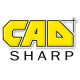## Get start and end points of sketch segment

You need to be logged in to view this content. Please login or become a member.

1.edgarquintana

Can you help me understand

vCurveParams(3)
vCurveParams(4)
vCurveParams(5)

?

API help says this values are direction.x, direction.y, direction.z, but i can’t find any sense to them.

I notice you used formula dblX = Round(vCurveParams(0) + dblLength * vCurveParams(3), 6) to calculate end point

How does it work?

•Keith

Edgar,

I am using vector algebra to calculate the end point since the end point values are not explicitly returned by ICurve::LineParams. The direction values refer to the vector. So to find the end point X coordinate, we take the start point X coordinate and add on the length times the X component of the line direction.

For example, let’s say we have a line that starts at (0, 0, 0)m and ends at (1, 0, 0)m. The length, of course, will be 1m. The direction vector will be (1, 0, 0) because all of the movement is in the X direction. So to calculate the end point coordinates we would do:

end pt X coord = start X + length * direction X = 0m + 1m * 1 = 1m
end pt Y coord = start Y + length * direction Y = 0m + 0m * 0 = 0m
end pt Z coord = start Z + length * direction Z = 0m + 0m * 0 = 0m

Therefore, the end pt of the line is (1, 0, 0)m.

•edgarquintana

In other words, direction vector is a coordinate in reference of the line star point, not the origin.
Is this statement correct?

If so, if we move the start point to (5,5,0) with a line length of 1m. the direction vector would
be again (1,0,0)m. and end point (6,5,0) Correct?

•Keith

Technically a vector is a magnitude (the distance) plus a direction. A direction isn’t a coordinate nor does it have any units; it doesn’t reference a point in space. The line could start anywhere, it will always have the same direction.

For a line with these characteristics.
Length = 1m
Direction = (1,0,0)
Start point: (5,5,0)m

…it is true that the end point will be (6,5,0)m.